Next: Axially Symmetric Cavities Up: Resonant Cavities and Waveguides Previous: Cavities with Rectangular Boundaries

# Quality Factor of a Resonant Cavity

The quality factor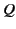of a resonant cavity is defined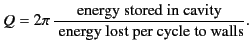(1320)

For a specific normal mode of the cavity, this quantity is independent of the mode amplitude. By conservation of energy, the power dissipated via ohmic losses is minus the rate of change of the stored energy,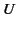. We can thus write a differential equation for the variation ofas a function of time: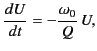(1321)

where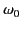is the oscillation frequency of the normal mode in question. The solution to the above equation is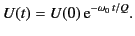(1322)

This time dependence of the stored energy suggests that the oscillations of the electromagnetic fields inside the cavity are damped as follows: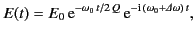(1323)

where we have allowed for a shift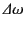of the resonant frequency, as well as for the damping. A damped oscillation such as that specified above does not consist of a pure frequency. Instead, it is made up of a superposition of frequencies centered on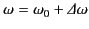. Standard Fourier analysis yields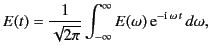(1324)

where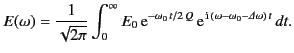(1325)

It follows that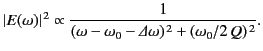(1326)

The above resonance curve has a full width at half-maximum equal to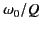. For a constant input voltage, the energy of oscillation within the cavity as a function of frequency follows this curve in the neighborhood of a particular resonant frequency. It can be seen that the ohmic losses, which determinefor a particular mode, also determine the maximum amplitude of the oscillation when the resonance condition is exactly satisfied, as well as the width of the resonance (i.e., how far off the resonant frequency the system can be driven, and still yield a significant oscillation amplitude).Next: Axially Symmetric Cavities Up: Resonant Cavities and Waveguides Previous: Cavities with Rectangular Boundaries
Richard Fitzpatrick 2014-06-27